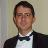# PVRP-DC instances

Published: 16 Aug 2018 | Version 1 | DOI: 10.17632/p4n2xw84bv.1
Contributor(s):Unspecified
• Juan-José Salazar-González,Mathematics
• Hande Yaman

## Description of this data

Here are the 240 instances used in the article "The Periodic Vehicle Routing Problem with Driver Consistency ", written by Inmaculada Rodríguez-Martín, Juan-José Salazar-González, and Hande Yaman, and published in "European Journal of Operational Research" 2018. The instance name gives information about the number of nodes, the number of time periods, and the number of vehicles. For example, test11-p2-m3-a-dat is an instance wiht 11 nodes, 2 time periods, and 3 vehicles.

These data files have the same format as the classical PVRP instances from the literature, though the vehicles' capacity and the customers' demands are not considered in this paper. That is:

The first line contains the following information:

type m n t

where

type = 1 (PVRP),
m = number of vehicles,
n = number of customers,
t = number of days.

The next t lines contain, for each day ,the following information:

D Q

where

D = maximum duration of a route (0 means 'unbounded'),
Q = maximum load of a vehicle,

The next lines contain, for the depot and each customer, the following information:

i x y d q f a list

where

i = customer number (0 corresponds to the depot),
x = x coordinate,
y = y coordinate,
d = service duration,
q = demand,
f = frequency of visit,
a = number of possible visit combinations,
list = list of all possible visit combinations.

Each visit combination is coded with the decimal equivalent of the corresponding binary bit string. For example, in a 5-day period, the code 10 which is equivalent to the bit string 01010 means that a customer is visited on days 2 and 4. (Days are numbered from left to right.)

## Experiment data files

• random instances
Cite

This data is associated with the following publication:

The periodic vehicle routing problem with driver consistency

• ### Version 1

2018-08-16

Published: 2018-08-16

DOI: 10.17632/p4n2xw84bv.1

### Cite this dataset

Rodriguez Martin, Inmaculada; Salazar-González, Juan-José; Yaman, Hande (2018), “PVRP-DC instances”, Mendeley Data, v1 http://dx.doi.org/10.17632/p4n2xw84bv.1

Views: 3908